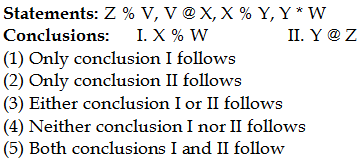## PREPARATORY QUESTIONS

### EQUAL-INEQUAL

#### PREPARATORY SET-65 (MODERATE)

Direction (Qs.1 to 5): In the following questions, the symbols @, #, %, \$ and * are used with the following meaning as illustrated below.

‘P @ Q’ means ‘P is neither greater than nor equal to Q‘

‘P % Q’ means ‘P is neither smaller than nor equal to Q’

‘P # Q’ means ‘P is not greater than Q’

‘P \$ Q’ means ‘P is not smaller than Q’

‘P * Q’ means ‘P is neither smaller than nor greater than Q’

Now in each of the following questions assuming the given statement to be true, find which of the two conclusions I and II given below them is/are definitely true and give your answer accordingly.

Question No : 1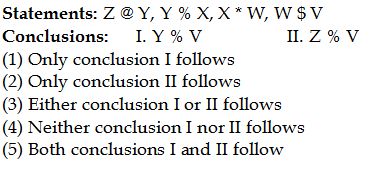Question No : 2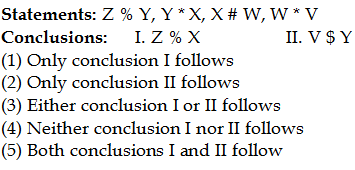Question No : 3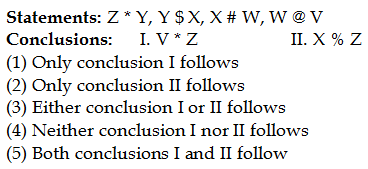Question No : 4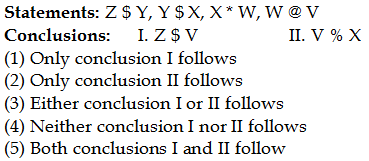Question No : 5